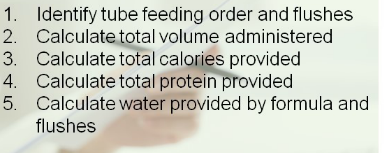# Tube feeding Flashcards

Set Details Share
created 8 years ago by bwilson
1,491 views
Page to share:
Embed this setcancel
COPY
code changes based on your size selection
Size:
X

1

Rate Based calculation stepsIdentify tube feeding order and flushes

calculate total calories provided

calculate total protein provided

calculate water provided by formula and flushes

2

Step 2: calculate total volume provided by formula

80 mL/hr x 24 hours= 1920 mL (1.92L)

1920 mL (1.92 L)

3

Step 3: calculate total calories provided by formula

1.92L x 1200 kcal/L=

2308 kcals

4

Step 4: calculate total protein provided by formula

1.92 L x 54 g protien=

104 g protein

5

step 5: calculate water provided by formula and water flushes

water in formula: 1920 mL x 0.81 = 1555 mL

water flushes: 100 mL x 6 flushes = 600 mL

total water provided: 1555 mL + 600 mL = 2155 mL total

...

6

Step 1. Identify tube feeding order and flushes

MD order: Fibersource HN at 80 mL/hr continuously with a 100 ML water flush Q4H.

(Note: one liter of Fibersource HN = 1200 k cal, 54 g protien, 81% free water)

Sample Rate- Based Calculation – based off nutritional requirements

1. Identify nutritional needs
2. Determine formula volume needed per day and rate
3. Calculate total calories provided
4. Calculate total protein provided
5. Calculate free water provided
6. Calculate additional water flushes needed
7. Write rate- based feeding order, including water flushes

7

Step 1. Identify nutrition needs

Assessment by RDN shows pt requires 1800 kcals, 70 g protein, and 1800 mL fluid. Pt requires standard polymeric formula.

2. Determine formula volume needed per day rate

nutren1.0 will be used (standard polymeric formula)

contains 1000 kcal, 40 g protien, and 83% free water per one liter

1800 kcal daily/ 1.0 kcal. mL= 1800 mL

1800 mL/24 hours per day = 75 mL/hr

8

3. calculate total calories provided

1800 mL daily x 1.0 kcal/mL = 1800 kcal daily

...

9

Calculate total protein provided

1.8 L (1800 mL) x 40 g protein/L = 72 grams

...

10

Calculate free water provided:

1800 mL x .83 = 1494 mL/d free water

...

11

total flush requirements = 1800 mL

1800 mL - 1494 mL free water = 306 mL needed

...

12

7. Write rate based feeding order, including water flushes

Nutren 1.0 at 75 mL/hr with a 150 mL water flush twice daily.

...

13

Bolus defined

a formula administered over a 5- 20 minute period using a syringe (60 mL syringe) several times each day

14

Bolus order components

(administer 240 mL of Nutren 1.5 Q4H with a 100 mL flush with each feeding)

Formula name

bolus volume

water flushes

time frame

15

inexpensive

mimics mealtime

portable

16

manual dexterity

higher risk of complications

17

We nourish website for bolus tube feedings or (syringe feeding)

must flush feeding tube with 1 oz of water or more

18

Sample Bolus calculation (steps)

(calculating a bolus feeding off of an existing order)

1.Identify tube feeding order and flushes

3. calculate total calories provided

4. calculate total protein provided

5. calculate water provided by formula and flushes

19

1.Identify tube feeding order and flushes

Physician orders "one can of nutren1.5 Q4H from 0800-2000,

Administer a 100 mL water flush with each feeding. " (Note: Nutren 1.5 contains 375 kcal/can, 17 g protein/ can and 76% free water)

20

2. Calculate total volume provided by formula

One can Q4H from 0800-2000 (8 am to 8 pm)

one can feeding at 8 am, 12 pm, 4 pm, 8 pm

each can of Nutern1.5 = 250 mL

Four cans daily = 1000 mL

21

3. Calculate total calories provided by formula

Calculate by cans: 250 mL can of Nutren 1.5 = 375 kcal/can x 4 cans = 1500 kcals

calculate by total volume: 250 mL can x 4 cans = 1000 mL x 1.5 kcal/mL = 1500 kcals

22

4. calculate total protein provided by formula:

calculate by cans:

calculate by cans: 250 mL can of Nutren 1.5 = 17 g pro/can x 4 cans = 68 g. protein

Calculate by total volume: 1000 mL = 68 g. protein

23

5. calculate water provided by formula and water flushes

water in formula: 250 mL can of Nutren 1.5 x 4 cans = 1000 mL x 0.76 (76% free water) = 760 mL free water

water flushes: 100 mL x 4 total feedings = 400 mL

total water provided: 760 mL + 400 mL = 1160 mL total

24

Sample Bolus Calculation:

Calculating a bolus feeding based off of nutritional requirements

1. Identify nutritional needs.

2. Determine number of cans per day

3. Calculate total calories per provided

4. Calculate total protein provided

5. Calculate free water provided

7. Write bolus feeding order, including water flushes

25

1. Identify nutritional needs.

Nutritional assessment by RDN reveals patient requires 2000 kcals, 80 g protein, and 1800 mL fluid

26

2. Determine formula and number of cans per day

A concentrated, 2.0 k cal/mL formula will be used - Nutren 2.0

One can of Nutren 2.0 = 500 kcals/can

2000 kcals required / 500 kcals per can = 4 cans daily

27

3. Calculate total calories provided

4 cans/day x 500 kcals daily = 2000 kcals

28

4. Calculate total protein provided

4 cans/day x 21 g protein = 84 g protein

29

5. Calculate free water provided

One can = 250 mL

4 cans/day x 250 mL = 1000 mL

69% free water per container in Nutren 2.0

1000 mL x 0.69 = 690 mL free water

30